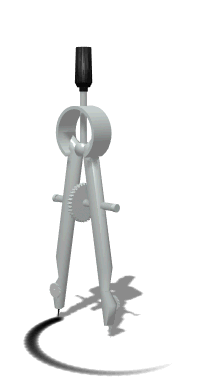•  2nd Benchmark Review

Units of Study
Divide Multi-Digit Numbers
Decimals- Add, Subtract, Multiply and Divide
Ratios
Rate and Speed
Percents
Factors and Multiples
Multiplication and Division of Fractions
Order of Operations
Algebraic Expressions
Algebraic Equations
Integers & Rational Numbers
Coordinate Graphing
Perimeter, Area, Surface Area & Volume
Statistics